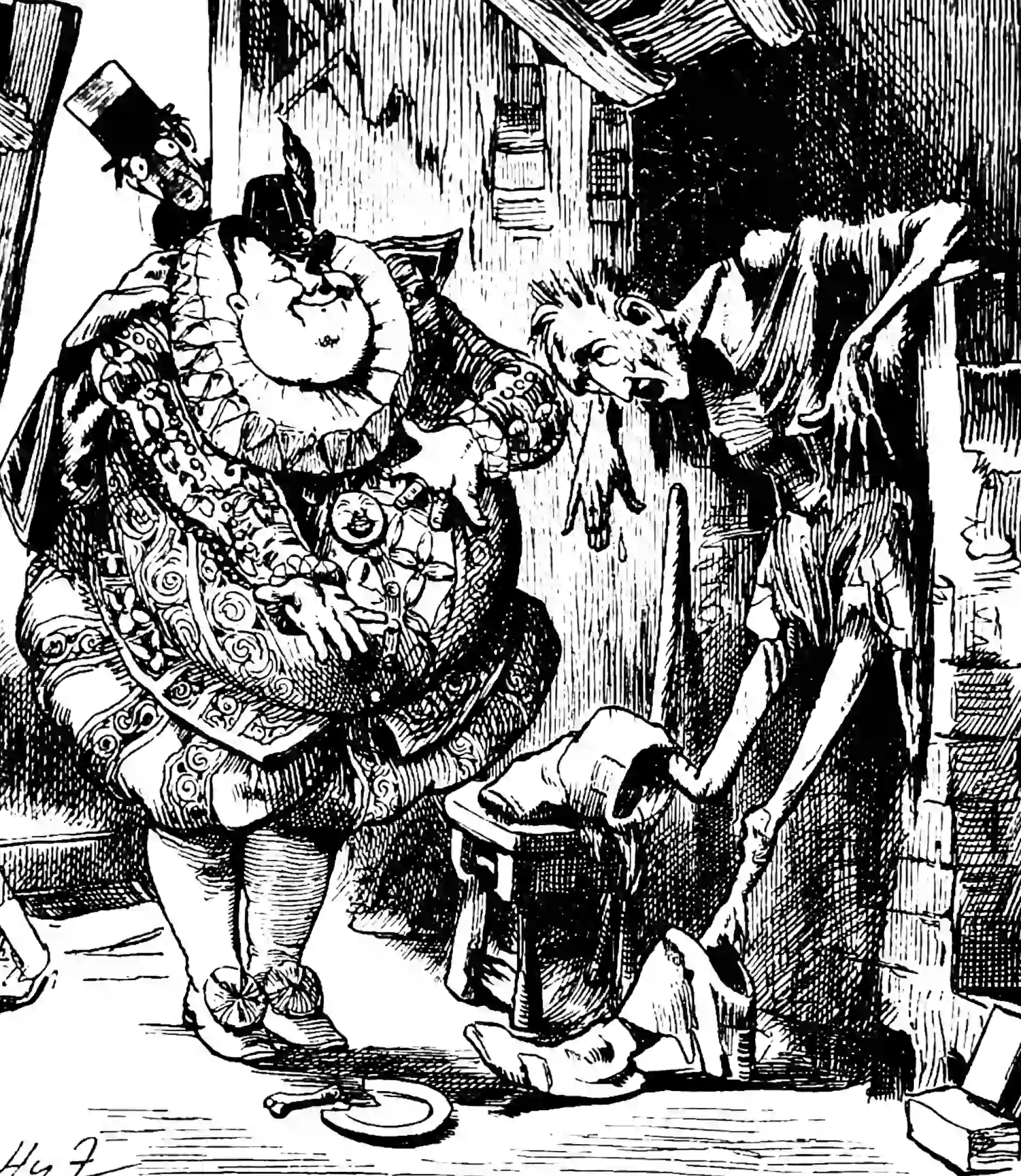# Gamma-Beta algebra

$\renewcommand{\var}{\operatorname{Var}} \renewcommand{\corr}{\operatorname{Corr}} \renewcommand{\dd}{\mathrm{d}} \renewcommand{\bb}{\mathbb{#1}} \renewcommand{\vv}{\boldsymbol{#1}} \renewcommand{\rv}{\mathsf{#1}} \renewcommand{\vrv}{\vv{\rv{#1}}} \renewcommand{\disteq}{\stackrel{d}{=}} \renewcommand{\gvn}{\mid} \renewcommand{\Ex}{\mathbb{E}} \renewcommand{\Pr}{\mathbb{P}}$Gamma (left) and Beta (right) processes.

An addendum to the gamma distribution notebook about some specialised results in Dufresne (1998). Everything here is transcribed from that paper with only light edits.

The main insight is that we can get a long way with the Mellin transform, $t \rightarrow \int_{\mathbb{R}} x^{t} \mu(d x)$ (Beware $$x^{t}$$ is $$x^{t-1}$$ in some papers.) Our results only require $$t \in \mathbb{R}$$, though the general theory of Mellin transforms is formulated for complex arguments.

The distribution with Mellin transform \begin{aligned} &\frac{\Gamma\left(a_{1}+t\right) \cdots \Gamma\left(a_{p}+t\right)}{\Gamma\left(a_{1}\right) \cdots \Gamma\left(a_{p}\right)} \times \frac{\Gamma\left(b_{1}\right) \cdots \Gamma\left(b_{q}\right)}{\Gamma\left(b_{1}+t\right) \cdots \Gamma\left(b_{q}+t\right)} \\ &\times \frac{\Gamma\left(c_{1}-t\right) \cdots \Gamma\left(c_{r}-t\right)}{\Gamma\left(c_{1}\right) \cdots \Gamma\left(c_{r}\right)} \times \frac{\Gamma\left(d_{1}\right) \cdots \Gamma\left(d_{s}\right)}{\Gamma\left(d_{1}-t\right) \cdots \Gamma\left(d_{s}-t\right)} \end{aligned} (if it exists) is denoted $\left(\begin{array}{ccc|ccc} a_{1} & \cdots & a_{p} & c_{1} & \cdots & c_{r} \\ b_{1} & \cdots & b_{q} & d_{1} & \cdots & d_{s} \end{array}\right) .$ The law exists, for instance, if $$p \geq q, a_{i}<b_{i}, 1 \leq i \leq q, r \geq s$$, $$c_{i}<d_{i}, 1 \leq i \leq s$$, in which case it is the same as the ratio of two independent variables with distributions $\beta_{a_{1}, b_{1}-a_{1}} \odot \cdots \odot \beta_{a_{q}, b_{q}-a_{q}} \odot \Gamma\left(a_{q+1}, 1\right) \odot \cdots \odot \Gamma\left(a_{p}, 1\right)$ and $\beta_{c_{1}, d_{1}-c_{1}} \odot \cdots \odot \beta_{c_{s}, d_{s}-c_{s}} \odot \Gamma\left(c_{s+1}, 1\right) \odot \cdots \odot \Gamma\left(c_{r}, 1\right)$

There are (at least) two useful rules captured by these symbols. The first is that the parameters which appear “above” and “below” may be cancelled, for instance if $$c_{1}=d_{2}$$ then the two parameters may be omitted.

The second is for the multiplication of independent variables: $\begin{gathered} \left(\begin{array}{ccc|ccc} a_{1} & \cdots & a_{p} & c_{1} & \cdots & c_{r} \\ b_{1} & \cdots & b_{q} & d_{1} & \cdots & d_{s} \end{array}\right) \odot\left(\begin{array}{ccc|ccc} a_{1}^{\prime} & \cdots & a_{p^{\prime}}^{\prime} & c_{1}^{\prime} & \cdots & c_{r^{\prime}}^{\prime} \\ b_{1}^{\prime} & \cdots & b_{q^{\prime}}^{\prime} & d_{1}^{\prime} & \cdots & d_{s^{\prime}}^{\prime} \end{array}\right) \\ =\left(\begin{array}{cccccc|cccccc} a_{1} & \cdots & a_{p} & a_{1}^{\prime} & \cdots & a_{p^{\prime}}^{\prime} & c_{1} & \cdots & c_{r} & c_{1}^{\prime} & \cdots & c_{r^{\prime}}^{\prime} \\ b_{1} & \cdots & b_{q} & b_{1}^{\prime} & \cdots & b_{q^{\prime}}^{\prime} & d_{1} & \cdots & d_{s} & d_{1}^{\prime} & \cdots & d_{s^{\prime}}^{\prime} \end{array}\right) . \end{gathered}$

TBC. For now, see the nifty application in Yor (2007).

### No comments yet. Why not leave one?

GitHub-flavored Markdown & a sane subset of HTML is supported.